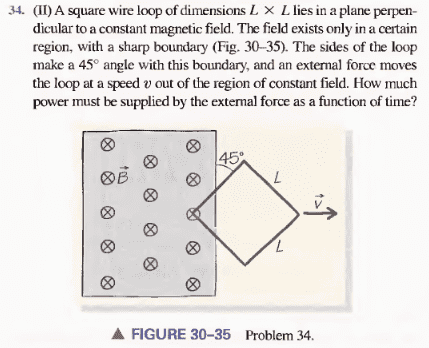# Motional EMF

## Homework Statement

I have the following problem for physics homework:We are supposed to take t=0 as the instant when the corner of the square is just about to leave the magnetic field.

Unsure.

## The Attempt at a Solution

Since the magnetic flux will decrease as the square leaves the field, the induced magnetic field because of the induced EMF in the square will also point downward. This tells us that the current will flow clockwise.

That's all I've got so far, and I don't even know if it's right. What is producing the forces here that would oppose our push on the square?

Edit: OK, I think that before tv=L/sqrt(2), power is 2IBtv^2. After tv = L/sqrt(2), power is 2IBv(sqrt(2)L - tv). Is it true that the current in the wire will change as the square gets pushed? If so, what do I do to figure out what current is in terms of the other variables?

Last edited: